Aller au contenu
Showing first {{hits.length}} results of {{hits_total}} for {{searchQueryText}}{{hits.length}} results for {{searchQueryText}}

Aucun résultat pour la recherche

(To write program code listings, please refer to this help page instead.) To typeset algorithms or pseudocode in LaTeX you can use one of the following options: Choose ONE of the (algpseudocode OR algcompatible OR algorithmic) packages to typeset algorithm bodies, and the algorithm package for captioning the algorithm. The algorithm2e package. Note that you should choose only one of the above groups of packages, and use only the commands and syntax provided by the package you choose. These packages cannot be loaded simultaneously; otherwise you will get lots of errors. Contents 1 The algpseudocode and algorithm packages 2 The algcompatible/algorithmic and algorithm packages 3 The algorithm2e package 4 Further Reading The algpseudocode and algorithm packages The algpseudocode package provides a algorithmic environment and some useful commands. You can  open a full example on Overleaf , and we'll go into some details in this section. Here's our first algorithm, using environments and commands from the algpseudocode package: \documentclass{article} \usepackage{algpseudocode} \begin{document} \begin{algorithmic} \State $i \gets 10$ \If{$i\geq 5$} \State $i \gets i-1$ \Else \If{$i\leq 3$} \State $i \gets i+2$ \EndIf \EndIf \end{algorithmic} \end{document}  Open this algpseudocode short example in Overleaf Here's the result output: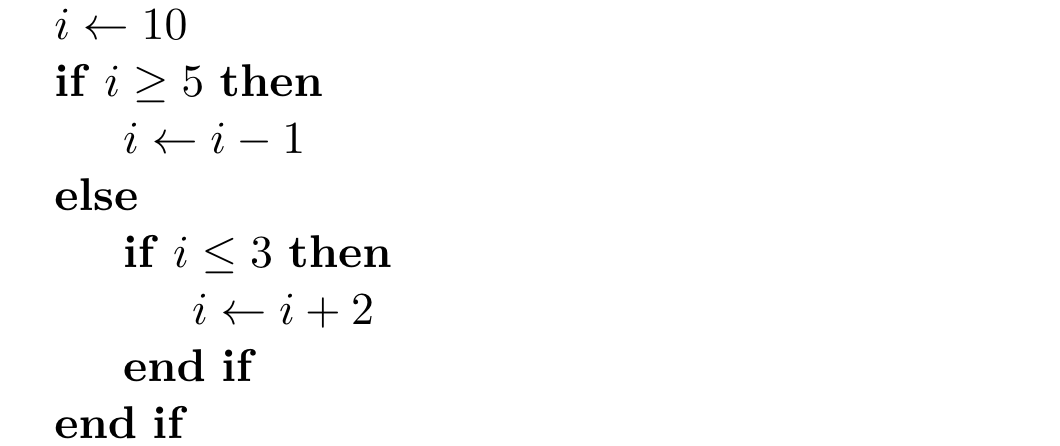You should not load the algorithm2e, algcompatible, algorithmic packages if you have already loaded algpseudocode. Note that the command names provided by algpseudocode are typically title-cased, e.g. \State, \While, \EndWhile. If you would like to add line numbers to the algorithm, you can add the first line number to the algorithmic environment like this: \begin{algorithmic} and get this output: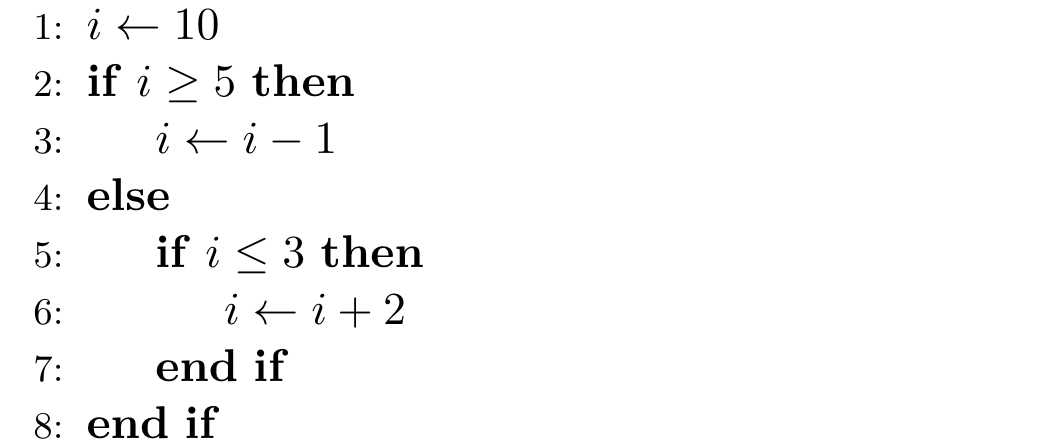The above algorithm example is not captioned nor numbered. If you need a captioned algorithm, you will also need to load the algorithm package, and add \begin{algorithm} \caption{...} ... \end{algorithm} around your algorithmic environment. You can use \label{...} after the \caption{...}, so that the algorithm number can be cross-referenced with \ref{...}. \documentclass{article} \usepackage{algorithm} \usepackage{algpseudocode} \begin{document} \begin{algorithm} \caption{An algorithm with caption}\label{alg:cap} \begin{algorithmic} \Require $n \geq 0$ \Ensure $y = x^n$ \State $y \gets 1$ \State $X \gets x$ \State $N \gets n$ \While{$N \neq 0$} \If{$N$ is even} \State $X \gets X \times X$ \State $N \gets \frac{N}{2}$ \Comment{This is a comment} \ElsIf{$N$ is odd} \State $y \gets y \times X$ \State $N \gets N - 1$ \EndIf \EndWhile \end{algorithmic} \end{algorithm} \end{document}  Open this algorithm+algpseudocode short example in Overleaf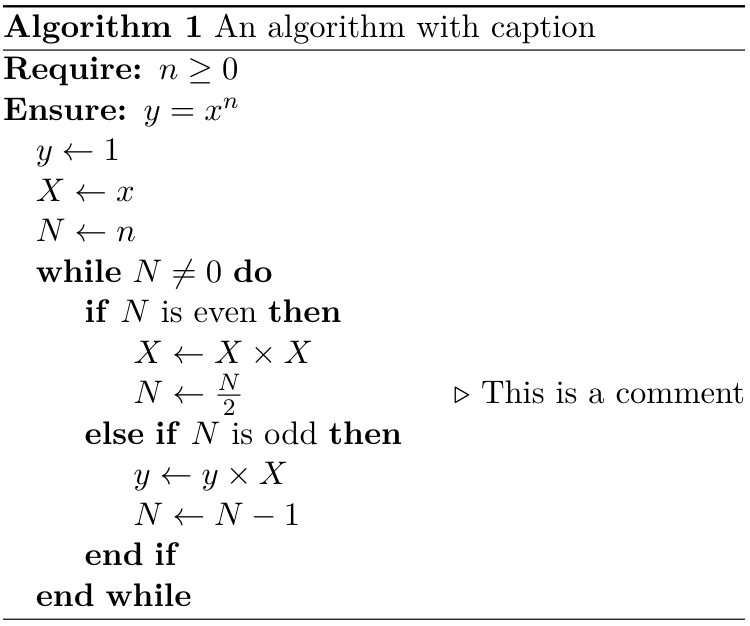The algorithm environment is a float like table and figure, so you can add float placement modifiers [hbt!] after \begin{algorithm} if necessary. This also means that while a long algorithmic environment on its own can break across pages, an algorithm environment won't. The algorithm package also provides a \listofalgorithms command that works like \listoffigures, but for captioned algorithms, like this.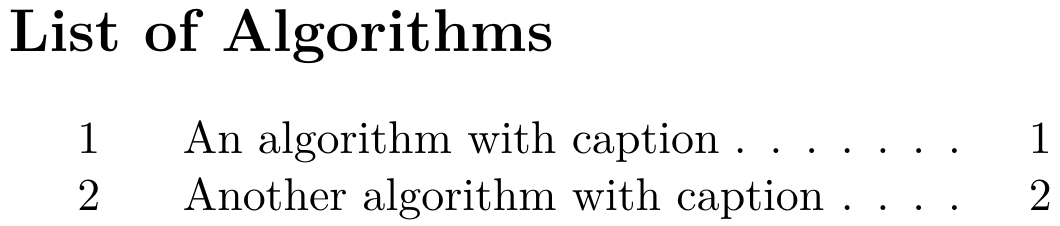Open a full example on Overleaf The algcompatible/algorithmic and algorithm packages The algorithmic package uses syntax similar to algpseudocode; but its command names are uppercased, e.g. \STATE, \WHILE, \ENDWHILE. On the other hand, algcompatible will recognise uppercased and title-cased command names, so \STATE, \WHILE, \ENDWHILE, \State, \While, \EndWhile are all recognised. Apart from the command names, algcompatible and algorithmic commands use the same arguments syntax as algpseudocode. \documentclass{article} \usepackage{algcompatible} % OR \usepackage{algorithmic} \begin{document} \begin{algorithmic} \STATE $i\gets 10$ \IF {$i\geq 5$} \STATE $i\gets i-1$ \ELSE \IF {$i\leq 3$} \STATE $i\gets i+2$ \ENDIF \ENDIF \end{algorithmic} \end{document}  Open this short algcompatible example in Overleaf Some older templates or document classes may have loaded algorithmic, so you will have to follow the syntax and command names provided. You should not load the algorithm2e, algpseudocode packages if the algorithmic or algcompatible package is already loaded. The algorithm package can be used with algorithmic/algcompatible to add numbered captions to the algorithms.  Open a full example on Overleaf The algorithm2e package The algorithm2e package has quite different syntax structure from the algpseudocode, algcompatible and algorithmic packages, so you will need to be careful about which package you want to use, or which package your template has loaded. The algorithm2e package provides an algorithm environment: \documentclass{article} \usepackage{algorithm2e} \begin{document} \begin{algorithm} $i\gets 10$\; \eIf{$i\geq 5$} { $i\gets i-1$\; }{ \If{$i\leq 3$} { $i\gets i+2$\; } } \end{algorithm} \end{document}  Open this short algorithm2e example in Overleaf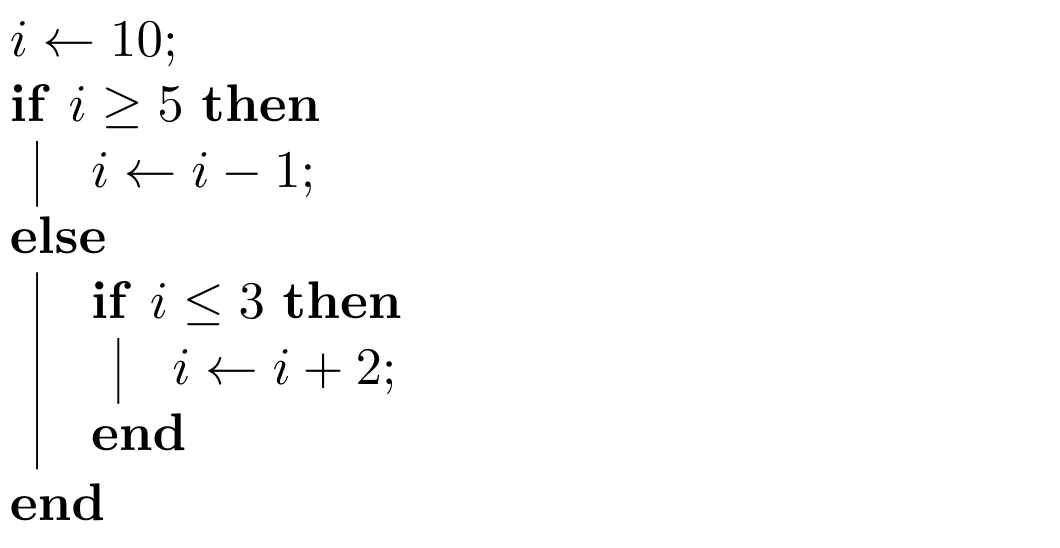Every line in your source code must end with \; otherwise your algorithm will continue on the same line of text in the output. Only lines with a macro beginning a block should not end with \;. When using algorithm2e you can use \caption{...}\ref{...} inside this algorithm environment directly, without needing to load any other packages. However if you want to add comments in your algorithm, you'll have to declare the command name to use first: %% This declares a command \Comment %% The argument will be surrounded by /* ... */ \SetKwComment{Comment}{/* }{ */} \begin{algorithm} \caption{An algorithm with caption}\label{alg:two} \KwData{$n \geq 0$} \KwResult{$y = x^n$} $y \gets 1$\; $X \gets x$\; $N \gets n$\; \While{$N \neq 0$}{ \eIf{$N$ is even}{ $X \gets X \times X$\; $N \gets \frac{N}{2}$ \Comment*[r]{This is a comment} }{\If{$N$ is odd}{ $y \gets y \times X$\; $N \gets N - 1$\; } } } \end{algorithm}  Open this captioned algorithm2e example in Overleaf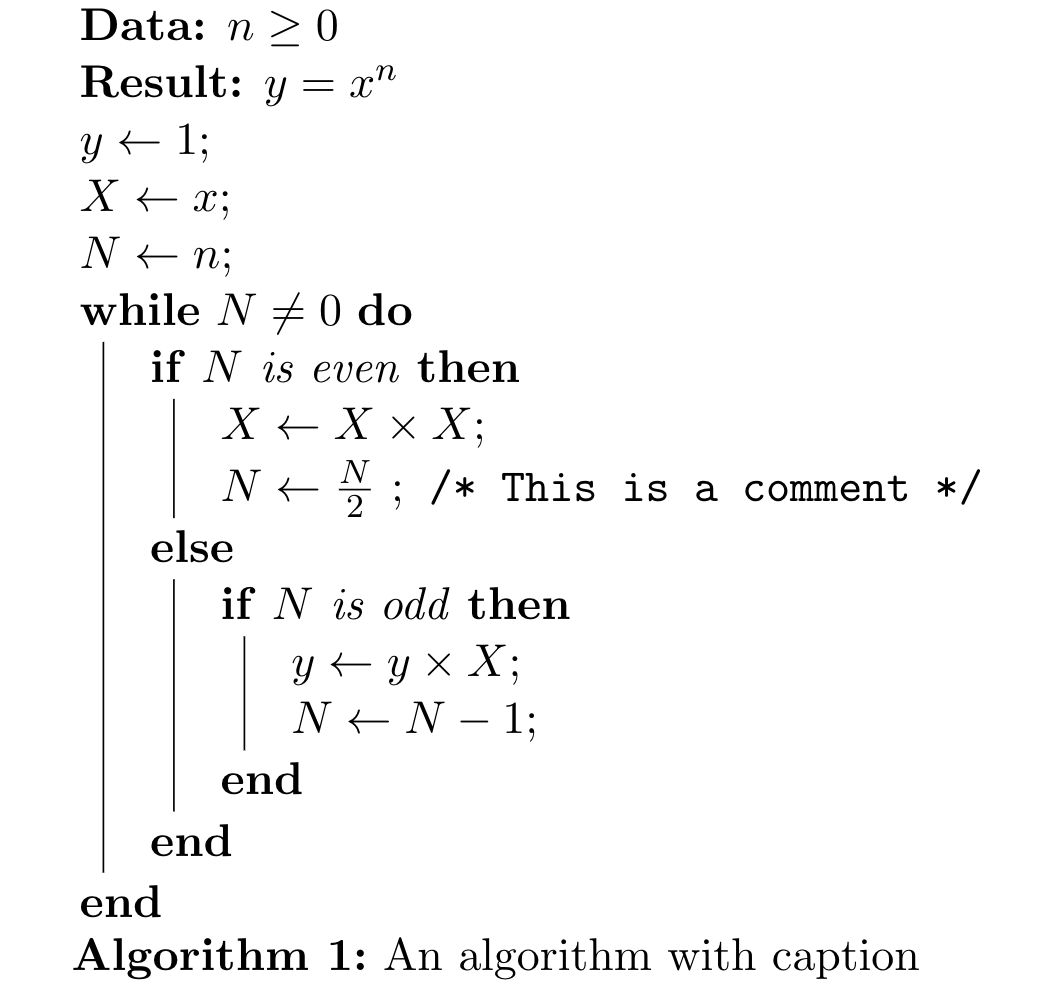By default, the plain algorithm style is used. But if you prefer lines around the algorithm and caption, you can add the ruled package option when loading algorithm2e, or write \RestyleAlgo{ruled} in your document. Your captioned algorithms will then be typeset like this: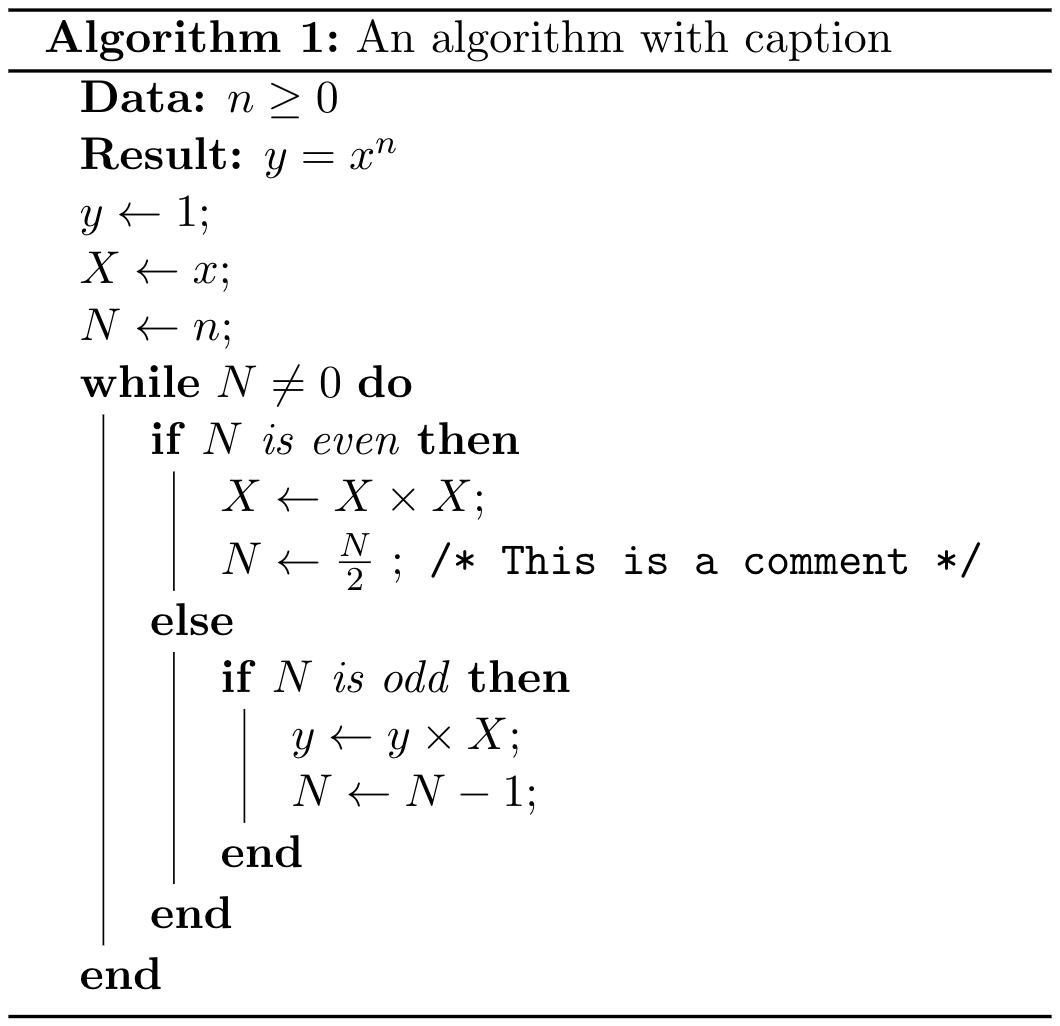The algorithm2e package provides many customisation options. For example, if you want to remove the vertical lines that mark the while—end while, if—end if blocks, you can add the noline package option when loading algorithm2e, or write \SetNoline. A \listofalgorithms command is also available in algorithm2e.  Open a full example on Overleaf Further Reading algpseudocode package documentation (see section 3.1) algorithmic and algorith package documentation algorithm2e package documentation